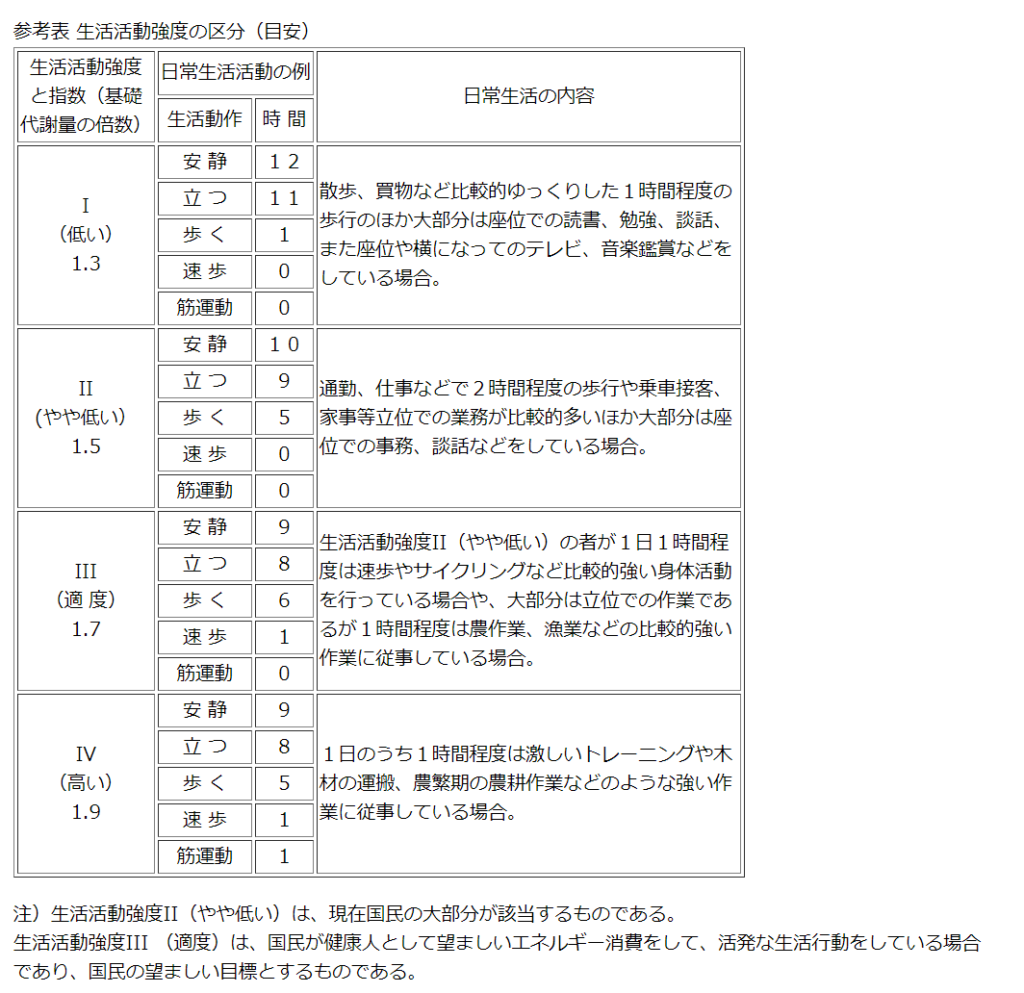Language Switcher

# Diet [Part 2] Know the daily calorie consumption.

Calorie adjustment is the basis for dieting (removing fat).

You can lose weight by (calorie consumption)> (calorie intake).

The calorie intake can be calculated by referring to the labeling of foods, but the calorie consumption varies from person to person, so you must calculate it yourself.

There are many ways to calculate this calorie burn, including one for athletes and one that is based on daily weight training bodybuilders.

Since it is difficult to check all of them, I will teach you the calculation method that succeeded in reducing the body fat percentage from 35% to 10% in one year.## Calculation of calories burned

Calories burned is calculated by multiplying basal metabolism (basal energy expenditure) by the daily activity intensity index.

Calculation formula: Calories burned = Basal metabolism (BMR) x Life intensity index

### What is Basal Metabolism (BMR)?

Basal metabolism is the minimum energy required to sustain life, and is the energy consumed without doing anything.

As a guide only, the daily metabolic rate of general adults is about 1,200 kcal for women and about 1,500 kcal for men, and it is said that the consumption is 27% for the liver, 19% for the brain, and 18% for the muscles. I will.

Basal metabolism that is indispensable for maintaining life such as the liver and brain.
No matter how much you want to lose weight, calorie restriction below basal metabolism is dangerous.

#### Calculation of basal metabolism (BMR)

The calculation method of basal metabolism includes “Harris-Benedict equation” and “Catch Macardle”.

Since the Harris-Benedict equation does not consider the body fat percentage, the value is calculated less for muscular people and more than the actual value for people with a lot of fat, so the formula considering the body fat percentage, Use “Catch Macardle”.

Calculation formula (catch macardle): Basal metabolism = 370 + 21.6 x lean body mass

Lean body mass is the weight of your body weight minus fat.

* Before actually calculating, please measure your body fat percentage using a scale that can measure your body fat percentage.

Calculation formula: Lean body mass = body weight x ((100-body fat percentage) / 100)

[Calculation example 1]

If you weigh 70 kg and have a body fat percentage of 18%

Lean body mass = 70 x ((100-18) / 100) = 57.4 (kg)

Basal metabolism = 370 + 21.6 x 57.4 (lean body mass) = 1,610 (kcal)

[Calculation example 2]

If you weigh 70 kg and have a body fat percentage of 25%

Lean body mass = 70 x ((100-25) / 100) = 52.5 (kg)

Basal metabolism = 370 + 21.6 x 52.5 (lean body mass) = 1,504 (kcal)

In this way, you can see that the basal metabolism rises in proportion to the amount of muscle mass.

### Life intensity index

The calories burned in a day differ greatly between those who usually work in the office and those who work hard, such as athletes.

This is quantified in the “Life Intensity Index” (table below). Then, the calorie consumption is calculated by multiplying this “life intensity index” by the basal metabolism (BMR).

Introduction of the site of “Ministry of Health, Labor and Welfare” in Japan.
第6次改訂日本人の栄養所要量　（厚生労働省）[Calculating based on a calculation example of basal metabolism]

For a person weighing 70 kg and having a body fat percentage of 18%, the basal metabolism was 1,610 (kcal), so

Multiply this by the “Life Intensity Index (III)” (people who are exercising moderately) to get the calories burned.

Calculation formula (example): 1,610 x 1.7 = 2,737 (kcal)

This is the daily calorie consumption of a person with a body weight of 70 kg and a body fat percentage of 18%, which is the standard for calorie intake without gaining weight or losing weight.

## To summarize the daily calorie consumption calculation

1. Measure body weight and body fat percentage.
2. Calculate lean body mass.
Calculation formula: Lean body mass = body weight x ((100-body fat percentage) / 100)
3. Calculate basal metabolism.
Calculation formula: Basal metabolism = 370 + 21.6 x lean body mass
4. Check the life intensity index (I-IV in the table) 5. Calculate calories burned.
Calculation formula: Calories burned = Basal metabolism (BMR) x Life intensity index

If you don’t know your calories burned, you can’t decide how much to lose weight, so please understand it well.

This time, it was how to calculate my daily calorie consumption.

Diet series [Part 1] to [Part 5]
I have an article about the specific calculation method required for dieting, so please read it in order!

Diet [Part 1] Not weight loss! Remove fat
Diet [Part 2] Know the daily calorie consumption
Diet [Part 3] Adjust calorie intake with three major nutrients
Diet [Part 4] How to eat
Diet [Part 5] Prepare the intestinal environment

muscle-training-dietblog fudousin